# Venn Diagrams and the Overlapping Set Equation

A Venn diagram or set diagram is a diagram that shows all possibilities of overlap and non-overlap of two or more sets. The simplest and most typical Venn diagram depicts two overlapping circles: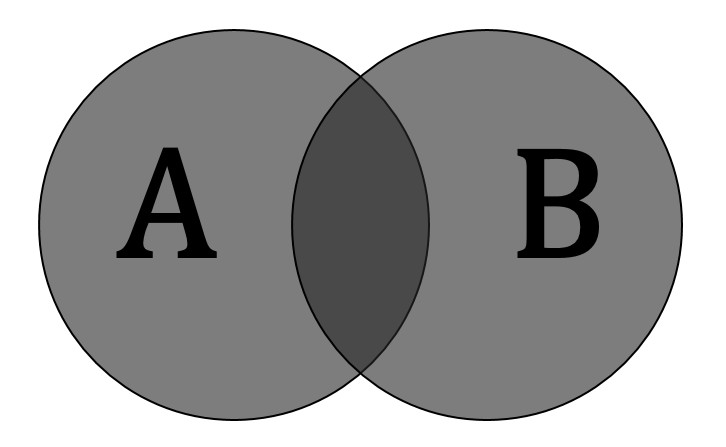Sets A (creatures with two legs) and B (creatures that can fly)

This example involves two sets, A and B, represented here as circles. Set A represents all living creatures that are two-legged. Set B represents the living creatures that can fly. Each separate type of creature can be imagined as a point somewhere in the diagram. Living creatures that both can fly and have two legs – for example, parrots – are in both sets, so they are represented by the area where the circles overlap.

Humans and penguins are bipedal, and so are then in Set A, but since they cannot fly, they appear in the left part of the left circle, where it does not overlap with the right circle. Mosquitoes have six legs, and fly, so the point for mosquitoes is in the part of the right circle that does not overlap with the left one. Creatures that are not two-legged and cannot fly (for example, whales and spiders) would be represented by the area outside both circles.

The combined area of sets A and B is the union of A and B, as we discussed in the previous section. The union in this case contains all living creatures that are either two-legged or that can fly or both. Moreover, the area in both A and B, where the two sets overlap, is called intersection of A and B, as discussed in the previous section. For example, the intersection of the two sets is not empty, because there are points that represent creatures that are in both circles.

The key characteristic of a Venn diagram is that it represents exhaustive possibilities. In other words, all the possible logical relations of these classes can be indicated in the same diagram. That is, the diagram initially leaves room for any possible relation of two sets, and the actual or given relation can then be specified by indicating that some particular region is null or is not-null.

Here are some of those “regions” that are null or not null in different cases, as shaded in different diagrams.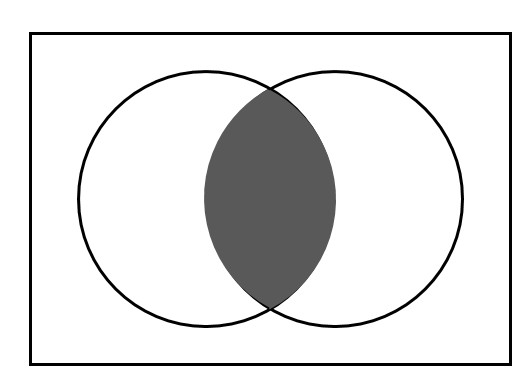Intersection of two sets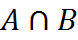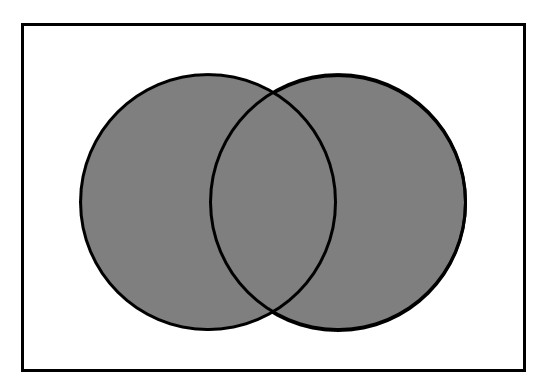Union of two sets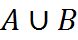## The Overlapping Set Equation

The overlapping set equation is tremendously important on the GMAT. To write this equation, we draw the Venn diagram inside a box, as in the latter two figures above. This is the lesser-known but superior way to draw a Venn diagram, because it clearly defines the region of things that are members of neither set – inside neither circle.

Returning to the first example,

the shaded area is the intersection of the two sets, the overlap of the two circles. We can introduce a simpler notation. Call the two sets G1 and G2, for Group 1 and Group 2, respectively. The shaded area is B, for “both” – it includes the elements that are in both sets. Finally, there is the area around the two circles (but inside the box). That’s N, for “neither” – it includes the elements that are not in either set.

(In our new notation, we are using the variables to stand for the counts of how many elements are in each set. So G1 stands for what we would write precisely in set notation in vertical bars. This is fine on the GMAT, because we don’t have to write formal set equations.)

If we add everything up, we get that the total number of elements here is G1, plus G2, plus N. But since G1 and G2 are overlapping, the region B – the shaded region – is counted twice. So, to count the total number accurately, we must subtract B once from that sum. Therefore,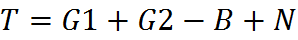This is the ever-so-useful Overlapping Set Equation. You can remember it and make use of it by drawing the Venn diagram above to jog your memory, if necessary. You are guaranteed to be able to use this equation on the GMAT. Whenever overlapping sets are in play, this equation is potentially useful.

## Three Overlapping Sets

Some more advanced GMAT questions concern three overlapping sets. The logic for three sets is similar to the logic for two sets, but the third set adds complexity to the situation.

Three overlapping sets can be depicted as follows: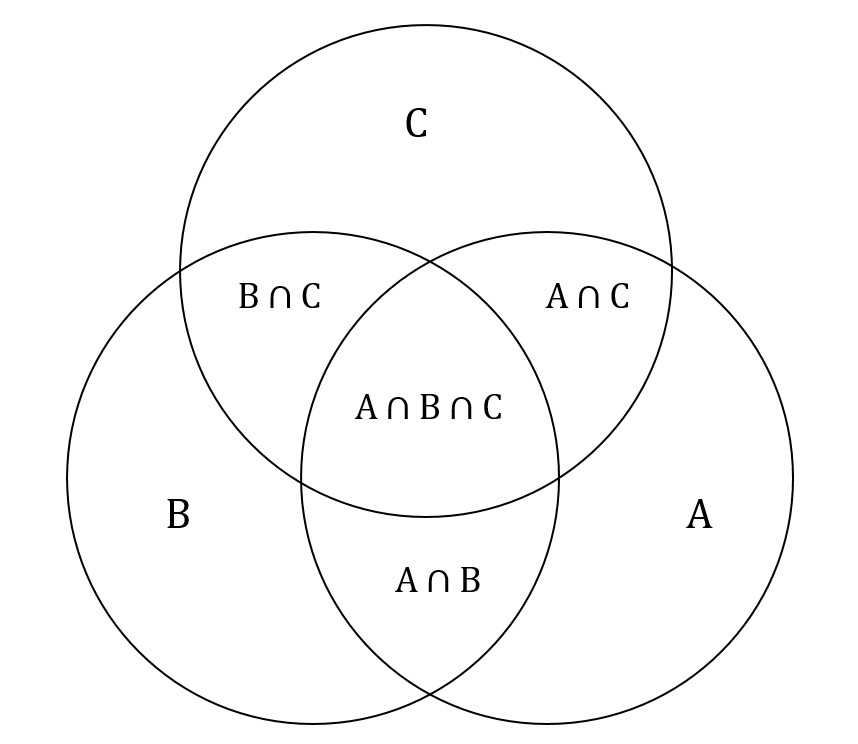And the overlap formula isHowever, as with two sets, we’ll write in an easier-to-remember notation, in just a moment. First, let’s look at this example: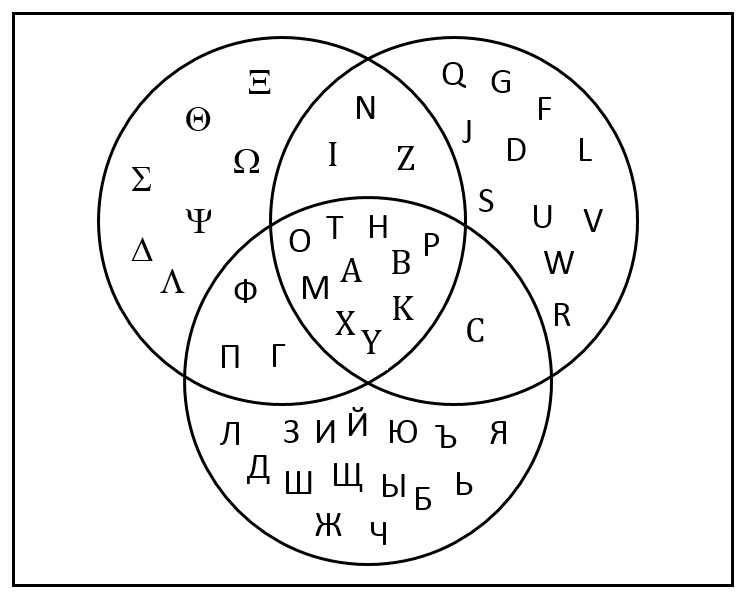This Venn diagram shows overlapping sets that contain letters. The upper-left circle contains the letters of the Greek alphabet, the upper-right circle contains the letters of the Latin alphabet, and the bottom circle contains the letters of the Russian alphabet.

Before, we had only one area of intersection, which we called “Both.” In the case of three sets, we have four areas of overlap. Three of them are logically similar in that they represent the overlap between two sets, whereas the section in the middle is the area of maximum overlap – all three sets. Namely, in the top overlap, N, Z, and I are all letters in both the Greek and the Latin alphabet, but not the Russian alphabet. And the surrounding box represents the universe of letters of alphabets. Some elements that would float in that area (but which are not depicted) are the letters of the Korean alphabet, none of which are members of the Greek, Latin, or Russian alphabets.

To add up all the elements of these sections to get the total number of elements, we employ the same logic as in the case of two sets and get: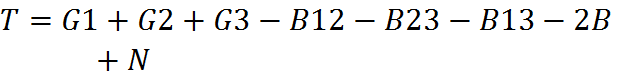As before, we must subtract to avoid double-counting – and in this case, triple-counting. If 1, 2, and 3 stand for Greek, Latin, and Russian, respectively, then B12, for example, would be the upper middle overlap portion, between 1 (Greek) and 2 (Latin), containing the letters N, Z, and I. We have counted it in G1 and again in G2, so we subtract it once in the equation above in order to count it a net of one time. Similarly, the middle section has been counted three times, one for each group, so we must subtract it twice in order to count it once ultimately.

As before, we can draw the Venn diagram in order to recall the formula for three overlapping sets. The key is remembering what we have to subtract and why in order to obtain the correct total number.